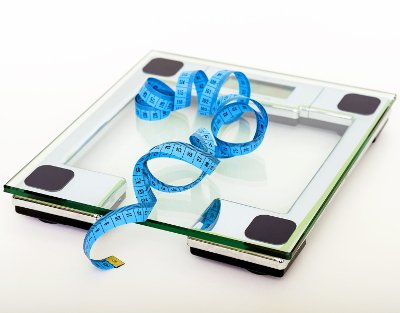# Total Body Water (TBW) Calculator

Share:

Total Body Water (TBW) is the total amount of water present in the body in the form of body fluids, tissues and organs. Here is an online calculator to find the quantity of water present in your body.

Please scroll down for details on using this Total Body Water calculator.

*
*
*
ft
Feet
in
Inches
cm
Centimeters
*
lb
Pounds
kg
Kilograms
*

Similar Fitness Calculators

## About Total Body Water (TBW) CalculatorThis online calculator is used to find the quantity of water present in human body.

### Using Total Body Water (TBW) calculator

3. Enter your height either in centimeters or in feet and inches.
4. Then, enter your weight either in kilograms or pounds.
5. Choose one of the formulas to calculate. Currently we are providing options to choose Watson Formula or Hume-Weyer Formula.
6. Finally, click the Calculate TBW button. The total body water will be calculated with your chosen formula, and the result will be displayed in the box below the button.

## Total Body Water (TBW)

Total Body Water (TBW) is the total amount of water present in the body in the form of body fluids, tissues and organs. In a broad way, water content is a human body is divided into two types; intracellular fluid and extracellular fluid. Intracellular fluid is the water stored inside the cells. It constitutes about two third of the total body water content. Extracellular fluid is the water stored and flowing outside the cells. Extracellular fluid constitutes about one third of the total body water. An average female adult human body contains 50% of water and a male adult body contains 60% of water. But this amount varies based on various factors like height, weight, age, disease, etc...

There are several formulas to calculate the total body water content. The popular and most used ones are the formulas put forward by:

• Watson PE, Watson ID and Batt R called as Watson Formula (TBW-W).
• Hume R, Weyers E called as Hume-Weyer Formula (TBW-H).
• Chertow GM, Lazarus JM, Lew NL, Ma L, Lowrie EG calles as Chertow Formula (TBW-C).
Here are the formulas.

### Total Body Weight Formulas

#### 1. Watson Formula

TBW-W (for Male) = 2.447 - (0.09156 x A) + (0.1074 x ht) + (0.3362 x wt) litres

TBW-W (for Female) = -2.097 + (0.1069 x ht) + (0.2466 x wt) litres

Where:

• A = age in years.
• ht = Height in centimeters(cm).
• wt = Weight in kilograms(kg).

#### 2. Hume-Weyer Formula

TBW-H (for Male) = (0.194786 x H) + (0.296785 x W) - 14.012934

TBW-H (for Female) = (0.344547 x H) + (0.183809 x W) - 35.270121

Where:

• H = Height in centimeters(cm).
• W = Weight in kilograms(kg).

#### 3. Chertow Formula

TBW-C = H x (0.0186104 x W + 0.12703384) + W x (0.11262857 x M + 0.00104135 x A - 0.00067247 x W - 0.04012056) - A x (0.03486146 x M + 0.07493713) - M x 1.01767992 + D x 0.57894981

Where:

• A = age in years.
• H = Height in centimeters(cm).
• W = Weight in kilograms(kg).
• M = Male (Yes = 1, No = 0).
• D = Diabetes (Yes = 1, No = 0).